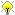Edwards coordinates formulas.

See email to sagedays6, along with http://hyperelliptic.org/EFD/edwards.html

Paul Zimmerman is working on this## First Formula

```%time
R.<y1,y2,c,d,x1,x2> = QQ[]
e = 1-d*c^4
S = R.quotient([x1^2+y1^2-c^2*(1+d*x1^2*y1^2), x2^2+y2^2-c^2*(1+d*x2^2*y2^2)])

x3 = (x1*y2+y1*x2)/(c*(1+d*x1*x2*y1*y2))
y3 = (y1*y2-x1*x2)/(c*(1-d*x1*x2*y1*y2))

# map to the Montgomery curve:
u1 = (c+y1)/(c-y1); v1=2*c*u1/x1
g = (1/e)*v1^2-u1^3-(4/e-2)*u1^2-u1
print S(g.numerator())

u2 = (c+y2)/(c-y2); v2 = 2*c*u2/x2
g = (1/e)*v2^2-u2^3-(4/e-2)*u2^2-u2
print S(g.numerator())

u3 =(c+y3)/(c-y3); v3=2*c*u3/x3
g = (1/e)*v3^2-u3^3-(4/e-2)*u3^2-u3
print S(g.numerator())

# add on the Montgomery curve:
lambd = (v2-v1)/(u2-u1)
r3 = (1/e)*lambd^2-(4/e-2)-u1-u2; s3 =lambd*(u1-r3)-v1;

print S((u3-r3).numerator()), S((v3-s3).numerator())
///
0
0
0
0 0
CPU time: 0.06 s,  Wall time: 0.09 s```

## Second Formula

```#Here is the SAGE code corresponding to the 2nd version of the #addition.
#Paul
# 2007 Bernstein/Lange, 10M + 1S + 1C + 1D + 7add,
# strongly unified:

R.<c,d,X1,Y1,X2,Y2,Z1,Z2> = QQ[]
e = 1-d*c^4;
S = R.quotient([(X1^2+Y1^2)*Z1^2-c^2*(Z1^4+d*X1^2*Y1^2),
(X2^2+Y2^2)*Z2^2-c^2*(Z2^4+d*X2^2*Y2^2)])
x1 = X1/Z1; y1 = Y1/Z1;
x2 = X2/Z2; y2 = Y2/Z2;
x3 = (x1*y2+y1*x2)/(c*(1+d*x1*x2*y1*y2));
y3 = (y1*y2-x1*x2)/(c*(1-d*x1*x2*y1*y2));

# here are the formulas:
A = Z1*Z2;
B = A^2;
C = X1*X2;
D = Y1*Y2;
E = d*C*D;
F = B-E;
G = B+E;
X3 = A*F*((X1+Y1)*(X2+Y2)-C-D);
Y3 = A*G*(D-C);
Z3 = c*F*G;

# check we get 0
print x3-X3/Z3
print y3-Y3/Z3```

## Third Formula

Here is another example, which was more tricky. David helped me. We had to use NumberField because QuotientRing did not work (unless I did a mistake).

Paul

```# 2007 Bernstein/Lange,
#    10M + 1S + 1C + 1D + 9add + 3timesi + 2times2,
# strongly unified:
# thanks to David Roe <[email protected]> for the help

Gauss.<i> = NumberField(x^2+1)
R.<c,d,X1,Y1,X2,Y2,Z1,Z2> = Gauss[]
e = 1-d*c^4
S = R.quotient([(X1^2+Y1^2)*Z1^2-c^2*(Z1^4+d*X1^2*Y1^2),
(X2^2+Y2^2)*Z2^2-c^2*(Z2^4+d*X2^2*Y2^2)])
x1 = X1/Z1; y1 = Y1/Z1
x2 = X2/Z2; y2 = Y2/Z2
x3 = (x1*y2+y1*x2)/(c*(1+d*x1*x2*y1*y2))
y3 = (y1*y2-x1*x2)/(c*(1-d*x1*x2*y1*y2))

# here are the formulas:
iX2 = i*X2;
C2 = Y2+iX2;
D2 = Y2-iX2;
iX1 = i*X1;
C1 = Y1+iX1;
D1 = Y1-iX1;
A = Z1*Z2;
B = 2*A^2;
C = C1*C2;
D = D1*D2;
L = D+C;
M = Y1*Y2;
N = 2*M-L;
E = d*M*N;
F = B-E;
G = B+E;
X3 = i*A*F*(D-C);
Y3 = A*G*L;
Z3 = c*G*F;
# check both are zero
print x3-X3/Z3
print y3-Y3/Z3```

days6/sprint/edwards (last edited 2008-11-14 13:42:05 by localhost)